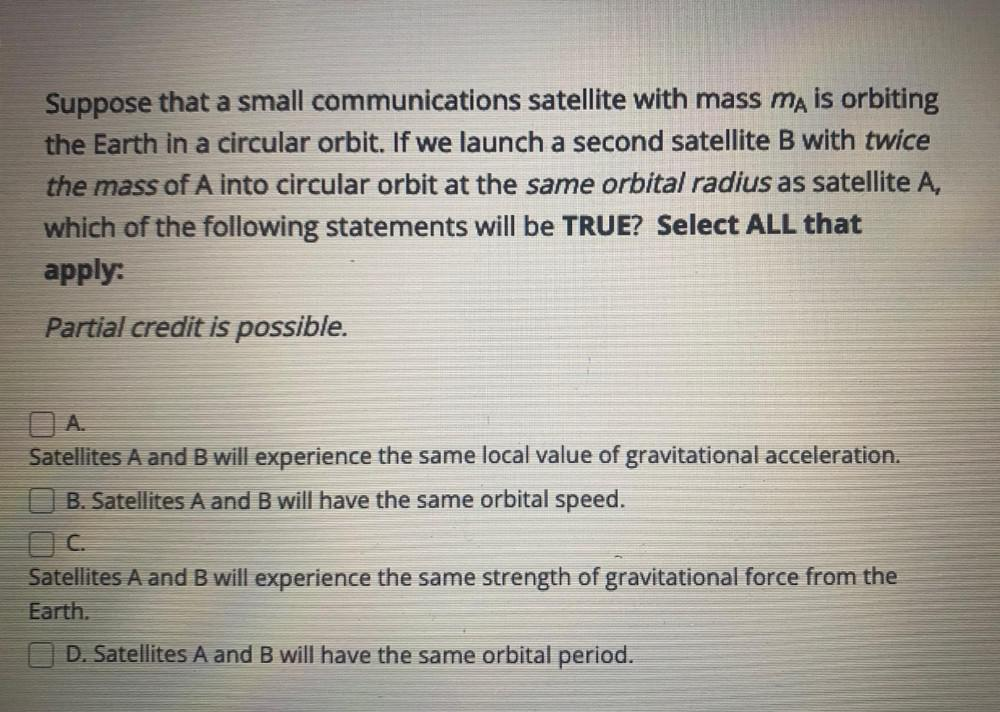Question:

# Suppose that a small communications satellite with mass mA is orbiting the Earth in a circular orbit. If we launch a second satellite B with twice the mass of A into circular orbit at the same orbitalSuppose that a small communications satellite with mass mA is orbiting the Earth in a circular orbit. If we launch a second satellite B with twice the mass of A into circular orbit at the same orbital radius as satellite A, which of the following statements will be TRUE? Select ALL that apply: (A) Satellites A and B will experience the same local value of gravitational acceleration. (B) Satellites A and B will have the same orbital speed. (C) Satellites A and B will experience the same strength of gravitational force from the Earth. (D) Satellites A and B will have the same orbital period.